NCERT Exemplar Solutions: Force & Laws of Motion

# NCERT Exemplar Solutions: Force & Laws of Motion - Science Class 9

## Multiple Choice Questions

Q.1. Which of the following statement is not correct for an object moving along a straight path in an accelerated motion?
(a) Its speed keeps changing
(b) Its velocity always changes
(c) It always goes away from the earth
(d) A force is always acting on it

Ans: c
Explanation:
To move away from earth Object’s acceleration should be more than the acceleration due to gravity. To escape from gravity only moving along a straight path is not enough hence option (c) is a wrong statement.
According to the third law of motion, action and reaction (a) always act on the same body (b) always act on different bodies in opposite directions (c) have the same magnitude and directions (d) act on either body at normal to each other.

Q.2. According to the third law of motion, action and reaction
(a) always act on the same body
(b) always act on different bodies in opposite directions
(c) have same magnitude and directions
(d) act on either body at normal to each other
Ans:
b
Explanation:
Newton’s third law states that “For every action, there is an equal and opposite reaction”. Hence answer is b)

Q.3. A goalkeeper in a game of football pulls his hands backwards after holding the ball shot at the goal. This enables the goal keeper to
(a) exert larger force on the ball
(b) reduce the force exerted by the ball on hands
(c) increase the rate of change of momentum
(d) decrease the rate of change of momentum
Ans:
b
Explanation:
Pulling hands backwards will help goalkeeper to reduce the momentum of the ball which in turn reduces the force of the ball exerted on goalkeeper’s hands.

Q.4. The inertia of an object tends to cause the object
(a) to increase its speed
(b) to decrease its speed
(c) to resist any change in its state of motion
(d) to decelerate due to friction
Ans:
c
Explanation:
Inertia is the property which resists the state of motion of an object. The object remains in its existing state of rest or uniform motion in a straight line unless that state is changed by an external force.

Q.5. A passenger in a moving train tosses a coin which falls behind him. It means that motion of the train is
(a) accelerated
(b) uniform
(c) retarded
(d) along circular tracks
Ans: a
Explanation:
If the motion of the train is uniform the coin would have fallen in his hand. If the motion is retarded coin would have fallen ahead of him. Since the coin falls behind the person motion of the train is accelerated.

Q.6. An object of mass 2 kg is sliding with a constant velocity of 4 m/s on a frictionless horizontal table. The force required to keep the object moving with the same velocity is
(a) 32 N
(b) 0 N
(c) 2 N
(d) 8 N
Ans:
b
Explanation:
Here the friction is opposing the force hence no force is required to keep the object in uniform motion. Hence, the answer is 0 N.

Q.7. Rocket works on the principle of conservation of
(a) mass
(b) energy
(c) momentum
(d) velocity
Ans:
c
Explanation:
The velocity of hot gases coming out of the rocket provided large momentum in the opposite direction which makes the rocket move upwards. Here the conservation of momentum takes place.

Q.8. A water tanker filled up to 2/3 of its height is moving with a uniform speed. On sudden application of the brake, the water in the tank would
(a) move backward
(b) move forward
(c) be unaffected
(d) rise upwards
Ans: b
Explanation:
On applying brakes water tanker comes to rest but water will be in motion this makes the water to come forward.

Q.9. There are three solids made up of aluminium, steel and wood, of the same shape and same volume. Which of them would have highest inertia?

Ans: Inertia depends on the mass of the object. For the solids with the same size and volume, inertia is determined by its mass. Hence steel will have the highest inertia.

Q.10. Two balls of the same size but of different materials, rubber and iron are kept on the smooth floor of a moving train. The brakes are applied suddenly to stop the train. Will the balls start rolling? If so, in which direction? Will they move with the same speed? Give reasons for your answer.
Ans:
Yes, the balls start rolling in the direction of the train’s movement. When brakes are applied, the train will come to rest and balls try to attain rest because of inertia balls remain in motion and they begin to roll. Since the mass between two balls is not the same, the inertia of the iron ball is greater than the inertia of the rubber ball. Hence, rubber ball rolls faster than the iron ball.

Q.11. Two identical bullets are fired one by a light rifle and another by a heavy rifle with the same force. Which rifle will hurt the shoulder more and why?

Ans:
The momentum of the bullet depends on the backward momentum of the rifle. As per Newton’s third law of motion: recoil velocity is equivalent to the bullet’s velocity, making it lighter. Since the momentum of the lighter rifle is more than the heavier rifle, the bullet fired from lighter rifle hurts the shoulder more.

Q.12. A horse continues to apply a force in order to move a cart with a constant speed. Explain why?

Ans:
When cart moves on the road it has to encounter friction. In order to keep the cart moving horse should overcome the friction. Hence it continues to apply the force.

Q.13. Suppose a ball of mass m is thrown vertically upward with an initial speed v, its speed decreases continuously till it becomes zero. Thereafter, the ball begins to fall downward and attains the speed v again before striking the ground. It implies that the magnitude of initial and final momentums of the ball are same. Yet, it is not an example of conservation of momentum. Explain why?
Ans:
If the external force did not act on the system, the momentum of that system remains constant. In the given case, change in velocity is due to the gravitational force of the earth and hence is not an example of conservation of momentum.

Q.14. Velocity versus time graph of a ball of mass 50 g rolling on a concrete floor is shown in Figure. Calculate the acceleration and frictional force of the floor on the ball.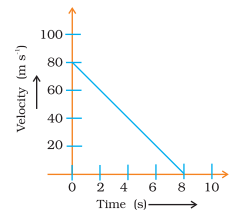Ans:
Given, m = 50g
F =?
Force = mass × acceleration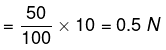velocity = 80ms-1
Velocity decelerates due to the friction of the floor with itself and comes to rest after 8 seconds.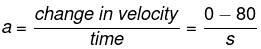= -10m/s2
The negative sign indicates that the frictional force exerted opposes the motion of the ball. Now, using Newton’s relation,
Force = mass× acceleration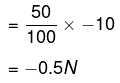Q.15. A truck of mass M is moved under a force F. If the truck is then loaded with an object equal to the mass of the truck and the driving force is halved, then how does the acceleration change?
Ans:

Force = mass x acceleration

F=ma

a= f/m

When mass is doubled force is halved m becomes 2m and f becomes f/2

a2=f/4m or a2/a1 = f/4m + f/4m = 1/4

acceleration reduces to 1/4th

Q.16. Two friends on roller-skates are standing 5 m apart facing each other. One of them throws a ball of 2 kg towards the other, who catches it, How will this activity affect the position of the two? Explain your answer.
Ans:

Distance between them will increase. Before throwing the ball, the momentum of both of them will be zero. To conserve the momentum, the person who throws the ball moves backwards. The person who catches the ball experiences a net force while catching the ball and he moves backwards.

Q.17. Water sprinkler used for grass lawns begins to rotate as soon as the water is supplied. Explain the principle on which it works.
Ans:
Water sprinkler works on the third law of motion. When water comes out of sprinkler an equal and opposite force is exerted. This will cause the sprinkler to rotate.

Q.18. Using second law of motion, derive the relation between force and acceleration. A bullet of 10 g strikes a sand-bag at a speed of 103 m/s and gets embedded after travelling 5 cm. Calculate
(i) the resistive force exerted by the sand on the bullet
(ii) the time taken by the bullet to come to rest.
Ans:

(i) m =10 g
= 10/1000kg u
= 103 m/s
v = 0
s= 5/100m
v2-u2= 2a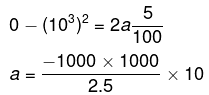=107ms-2
F = ma
=105N

(ii) v=u+at
0=103-107t
107t= 103
t=103/107
= 10-4s

Q.19. Derive the unit of force using the second law of motion. A force of 5 N produces an acceleration of 8 ms-2 on a mass m1 and an acceleration of 24 ms-2 on a mass m2. What acceleration would the same force provide if both the masses are tied together?
Ans:

F = m a = kg ms–2
This unit is also called newton. Its symbol is N.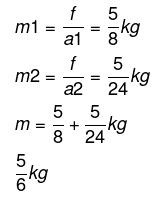Acceleration produced in M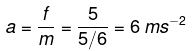Q.20. What is momentum? Write its SI unit. Interpret force in terms of momentum. Represent the following graphically
(a) momentum versus velocity when mass is fixed.
(b) momentum versus mass when velocity is constant.
Ans:
The quantity of motion of a moving body is called momentum. It is measured as product of mass and velocity.
Momentum = mass × velocity
SI unit of momentum is kg m s–1
Force = Rate of change in momentum

1)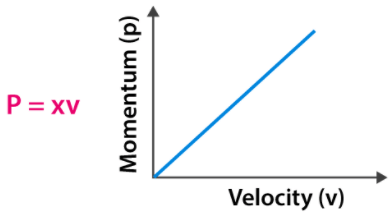2)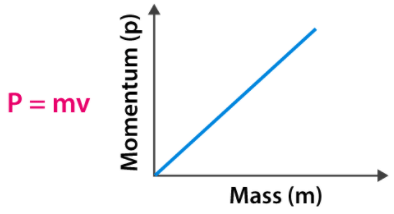The document NCERT Exemplar Solutions: Force & Laws of Motion | Science Class 9 is a part of the Class 9 Course Science Class 9.
All you need of Class 9 at this link: Class 9

## Science Class 9

66 videos|352 docs|97 tests

## FAQs on NCERT Exemplar Solutions: Force & Laws of Motion - Science Class 9

 1. What are the three laws of motion?Ans. The three laws of motion are: 1. Newton's First Law of Motion: An object at rest will remain at rest, and an object in motion will continue to move with a constant velocity unless acted upon by an external force. 2. Newton's Second Law of Motion: The rate of change of momentum of an object is directly proportional to the force applied, and it takes place in the direction in which the force acts. 3. Newton's Third Law of Motion: For every action, there is an equal and opposite reaction.
 2. What is the difference between mass and weight?Ans. Mass is the amount of matter present in an object, while weight is the force with which an object is attracted towards the center of the Earth (or any other celestial body). Mass is measured in kilograms, while weight is measured in Newtons.
 3. What is inertia?Ans. Inertia is the property of an object that resists any change in its state of motion. It is directly related to the mass of the object. Objects with greater mass have greater inertia, meaning they are harder to move or stop.
 4. What is the relationship between force, mass, and acceleration?Ans. According to Newton's second law of motion, the force acting on an object is directly proportional to its mass and the acceleration produced. Mathematically, it can be represented as F = m * a, where F is the force, m is the mass, and a is the acceleration.
 5. Can an object be at rest and still have forces acting on it?Ans. Yes, an object can be at rest and still have forces acting on it. According to Newton's first law of motion, an object at rest will remain at rest unless acted upon by an external force. So, even though the object is not moving, there may be forces acting on it that are balanced or canceled out, resulting in no net motion.

## Science Class 9

66 videos|352 docs|97 testsExplore Courses for Class 9 examSignup to see your scores go up within 7 days! Learn & Practice with 1000+ FREE Notes, Videos & Tests.
10M+ students study on EduRev
Track your progress, build streaks, highlight & save important lessons and more!
Related Searches

,

,

,

,

,

,

,

,

,

,

,

,

,

,

,

,

,

,

,

,

,

;